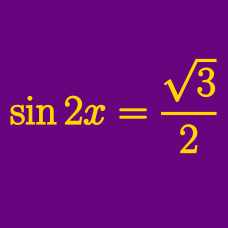Geometry

# Trigonometric Equations - Double Angle Formula

In the domain $[0^\circ , 1000^\circ ]$, how many solutions are there to $\sin \theta \cos \theta = \frac {1} {2}$?

In the interval $[0, 2 \pi ]$, how many solutions are there to

$\sin \theta = 2 \sin 2 \theta ?$

How many $x$'s in the interval $0 \leq x \leq 29\pi$ satisfy $\sin \left(2x-\frac{\pi}{5}\right)=\sin \frac{\pi}{8}?$

How many $x$'s in the interval $0 \leq x \leq 60\pi$ satisfy $6\cos 2x+23\sin x-4=0?$

In the interval $[0, 2 \pi ]$, how many solutions are there to

$\cos^2 \theta = \cos 2 \theta ?$

×